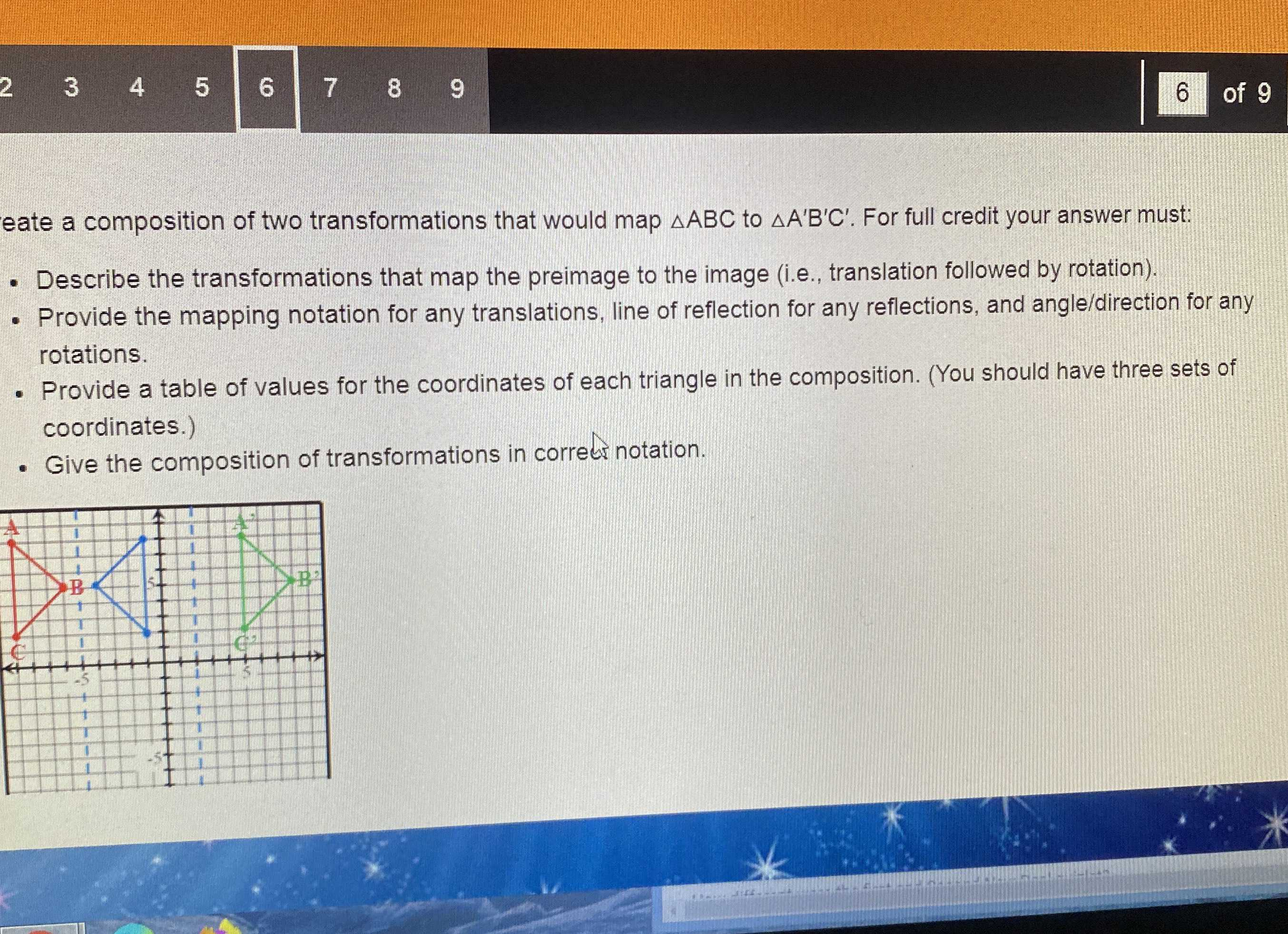### Still have math questions?

Trigonometry
QuestionCreate a composition of two transformations that would map $$\triangle A B C$$ to $$\triangle A ^ { \prime } B ^ { \prime } C ^ { \prime }$$ . For full credit your answer must:

Describe the transformations that map the preimage to the image (i.e., translation followed by rotation). - Provide the mapping notation for any translations, line of reflection for any reflections, and angle/direction for any rotations. - Provide a table of values for the coordinates of each triangle in the composition. (You should have three sets of coordinates.) - Give the composition of transformations in correcr notation.